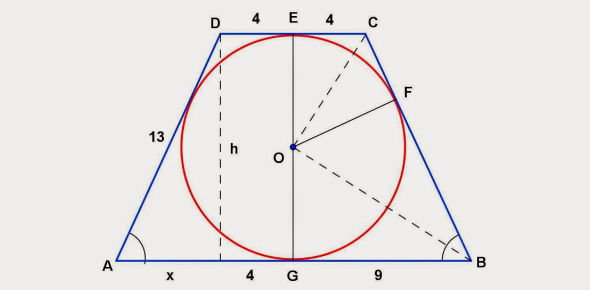# Area And Perimeter Of Trapezoids And Circles

13 Questions | Total Attempts: 483SettingsGeometry quiz on area and perimeter of trapezoids and circles

• 1.
Find the area of the trapezoid
• A.

2700

• B.

1350

• C.

435

• D.

217.5

• 2.
Find the area of the trapezoid.
• A.

65

• B.

130

• C.

720

• D.

360

• 3.
FInd the area of the trapezoid.
• A.

21.25

• B.

42.5

• C.

30

• D.

6.25

• 4.
Find the area of the trapezoid.
• A.

112

• B.

224

• C.

42

• D.

154

• 5.
Type in the perimeter in the box below for the trapezoid.
• 6.
Type in the perimeter in the box below for the trapezoid.
• 7.
Type in the perimeter in the box below for the trapezoid.
• 8.
Type in the area for the circle below. Use 3.14 for pi.
• 9.
Type in the area for the circle below. Use 3.14 for pi.
• 10.
Type in the area for the circle below. Use 3.14 for pi.
• 11.
Type in the circumference for the circle below. Use 3.14 for pi.
• 12.
Type in the circumference for the circle below. Use 3.14 for pi.
• 13.
Type in the circumference for the circle below. Use 3.14 for pi.
Related TopicsBack to top